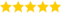# Java BASIC Programs

Last updated on 18th Sep 2020, Artciles, Blog

Kernel (Sr Project Manager )

He is a TOP-Rated Domain Expert with 6+ Years Of Experience, Also He is a Respective Technical Recruiter for Past 3 Years & Share's this Informative Articles For Freshers(5.0) | 17307 Ratings 637

Java is a programming language and computing platform first released by Sun Microsystems in 1995. There are lots of applications and websites that will not work unless you have Java installed, and more are created every day. Java is fast, secure, and reliable. From laptops to datacenters, game consoles to scientific supercomputers, cell phones to the Internet, Java is everywhere!

Java Basic Programs:

The following are some of the basic programs executed using java programming language.

1.Sum of two numbers

• {
• public static void main(String[] args)
• {
• int num1 = 5, num2 = 15, sum;
• sum = num1 + num2;
• System.out.println(“Sum of these numbers: “+sum);
• }
• }

Output:

Sum of these numbers: 20

2.Check Even or Odd number

• import java.util.Scanner;
• class CheckEvenOdd
• {
•  public static void main(String args[])
•   {
•  int num;
• System.out.println(“Enter an Integer number:”);
• //The input provided by user is stored in num
• Scanner input = new Scanner(System.in);
• num = input.nextInt();
• /* If number is divisible by 2 then it’s an even number
•  * else odd number*/
•  if ( num % 2 == 0 )
• System.out.println(“Entered number is even”);
• else
• System.out.println(“Entered number is odd”);
• }
• }

Output 1:

Enter an Integer number:

78

Entered number is even

Output 2:

Enter an Integer number:

77

Entered number is odd

3.Check whether the input year is leap or not

• import java.util.Scanner;
• public class Demo {
•  public static void main(String[] args) {
• int year;
• Scanner scan = new Scanner(System.in);
• System.out.println(“Enter any Year:”);
• year = scan.nextInt();
• scan.close();
•      boolean isLeap = false;
•  if(year % 4 == 0)
• {
•   if( year % 100 == 0)
•    {
•         if ( year % 400 == 0)
•             isLeap = true;
•       else
•              isLeap = false;
•    }
•    else
•              isLeap = true;
•   }
•  else {
•         isLeap = false;
•     }
•     if(isLeap==true)
•       System.out.println(year + ” is a Leap Year.”);
•   else
•      System.out.println(year + ” is not a Leap Year.”);
•  }
• }

Output 1:

Enter any Year:

2001

2001 is not a Leap Year.

Output 2:

Enter any Year:

2016

2016 is a Leap Year.

4.Check if the input string is palindrome or not.

• import java.util.Scanner;
• class PalindromeTest {
• public static void main(String args[])
• {
• String reverseString=””;
•     Scanner scanner = new Scanner(System.in);
•  System.out.println(“Enter a string to check if it is a palindrome:”);
•  String inputString = scanner.nextLine();
•   int length = inputString.length();
•      for ( int i = length – 1 ; i >= 0 ; i– )
•  reverseString = reverseString + inputString.charAt(i);
•  if (inputString.equals(reverseString))
•        System.out.println(“Input string is a palindrome.”);
•  else
•        System.out.println(“Input string is not a palindrome.”);
•  }
• }

Output 1:

Enter a string to check if it is a palindrome:

aabbaa

Input string is a palindrome.

Output 2:

Enter a string to check if it is a palindrome:

aaabbb

Input string is not a palindrome.

If you wanna use While Loop in above program then replace the for loop with this code:

• int i = length-1;
• while ( i >= 0){
•  reverseString = reverseString + inputString.charAt(i);
•  i–;
• }

To understand a programming language you must practice the programs, this way you can learn the language faster.

Are you looking training with Right Jobs?